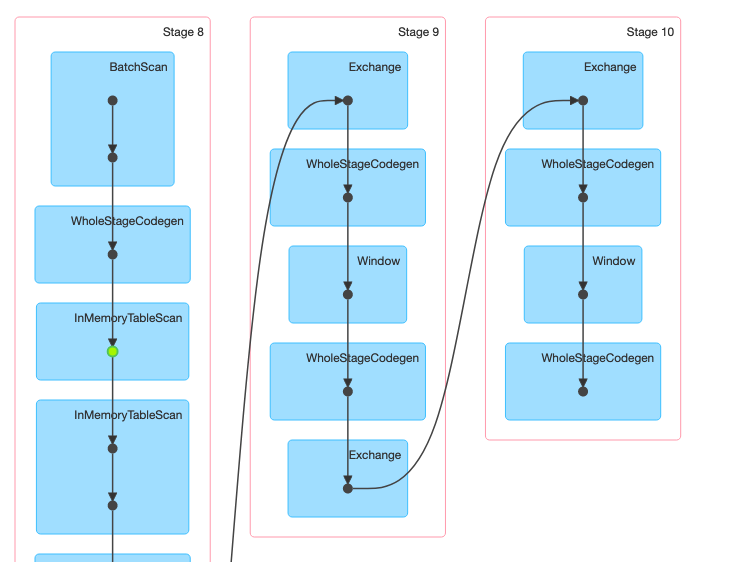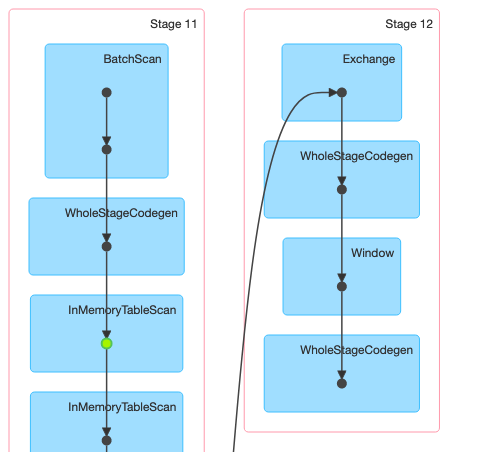Spark

# Remember to execute the windowing once with SparkMarti Sec
Apr 28, 2020

This is one of the things it makes sense when you stop to think about it. When you are performing the same aggregation over a window in more than one column, it is recommended to execute only once that windowing.

Lets dive in with an example: I am working with btc stock market data. What is called tick to tick, containing all the transactiom history. A common pattern in those type of analysis is to compare two consecutive values.

time price
1 2.2
1.2 2.1
1.8 2.4

Then, we shift the cells down for example, so we can do something like the following to obtain a metric called sojourn:
$T_i=t_i - t_{i-1}$

To do so, the most efficient way in spark is via row-by-row operation, but we must shift down the columns. An intermediate step would be the following:

time time_prev price price_prev
1 null 2.2 null
1.2 1 2.1 2.2
1.8 1.2 2.4 2.1

In spark we can do this via windowing functions applying the lag function to shift values down or the lead one if we want the reverse effect. If we just have one column to shift, there is no issue. However, if we have multiple of them, something common in those analysis, the first thing it may come to our mind is to do the following

import org.apache.spark.sql.expressions.Window

val previousRowSpec = Window.partitionBy(month('ts)).orderBy('time.asc).rowsBetween(-1, -1)

val multWindows = df.
withColumn("sojourn",'time - lag('time, 1).over(previousRowSpec)).
withColumn("X", 'price - lag('price, over(previousRowSpec))


Here we are executing twice the window, as we can see in the job DAG, we have two stages with its respective sort task (taking > 2 min in my dataset).The efficient thing to do here would be to execute the window only once. The trick is the struct() function.

val singleWindow = df.
withColumn("windowed_cols",lag(struct('time,'price),1).over(previousRowSpec)).
withColumn("sojourn", 'time - $"windowed_cols.time"). withColumn("X", 'price -$"windowed_cols.price").
drop('windowed_cols)And checking the times, we see an improvement with the second approach, as expected.

spark.time(multWindows.write.parquet("out1")) // 3 min 40sec
spark.time(singleWindow.write.parquet("out2")) // 2 min